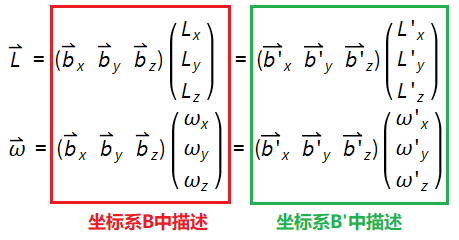# 刚体质量分布与牛顿-欧拉方程

## 惯性矩、惯性积、转动惯量、惯性张量

• 惯性矩是一个几何量，通常被用作描述截面抵抗弯曲的性质。惯性矩的国际单位为(m4)。即面积二次矩，也称面积惯性矩，而这个概念与质量惯性矩（即转动惯量）是不同概念。
面积元素dA与其至z轴或y轴距离平方的乘积y2dA或z2dA，分别称为该面积元素对于z轴或y轴的惯性矩或截面二次轴矩。惯性矩的数值恒大于零。对Z轴的惯性矩：$I_z=\int_A y^2 dA$，对Y轴的惯性矩：$I_y=\int_A z^2 dA$• 惯性积：质量惯性积是刚体动力学中一个重要的质量几何性质。刚体中的质量微元 Δmi与这微元的两个直角坐标的乘积对刚体的总和。其数值为：$$I_{xy}=\sum_{i}m_ix_iy_i \quad or \quad I_{xy}=\int xydm$$

对于给定的物体，惯性积的值与建立的坐标系的位置及方向有关，如果我们选择的坐标系合适，可使惯性积的值为零。当对于某一坐标轴的惯性积为零时，这种特定的坐标轴称为惯性主轴或主轴（principal axes），相应的质量惯性矩称为主惯性矩（principal moments of inertia）。显然，如果刚体本身具有某种几何对称性，那么它的主轴方向总是沿着它的对称轴的。但是即使是完全没有任何对称性的刚体也是存在惯性主轴的。

• 转动惯量(Moment of Inertia)是刚体绕轴转动时惯性的量度。 在经典力学中，转动惯量又称质量惯性矩。对于一个质点，J = mr²，其中 m 是其质量，r 是质点和转轴的垂直距离。• 惯性张量(Inertia tensor)是描述刚体作定点转动时的转动惯性的一组惯性量，其表现形式为由9个分量构成的对称矩阵，刚体作定点转动的力学情况要比定轴转动复杂得多。惯性张量描述了物体的质量分布。以{A}为参考坐标系，刚体相对于坐标系{A}的惯性张量用3×3的矩阵表达：惯性张量矩阵的特征值是主惯性矩，其对应的特征向量是惯性主轴的方向向量The eigenvalues of an inertia tensor are the principal moments for the body. The associated eigenvectors are the principal axes.） 已知某参考坐标系下的惯性张量，要计算惯性主轴可以参考

## 同一向量在不同参考系中的描述

考虑2个与刚体固连的坐标系$B$和$B'$，其中坐标系$B$的基向量为${\vec{b_x},\vec{b_y},\vec{b_z}}$，坐标系$B'$的基向量为${\vec{b_x'},\vec{b_y'},\vec{b_z'}}$。角动量及角速度在这两个坐标系中的描述为（The angular momentum vector and angular velocity vector of a rigid body are expressed in terms of these basis vectors, as follows ）：

##可以看出在不同坐标系下，描述同一个矢量的坐标是不一样的（因为参考基准不一样）。注意当我们提及“速度”、“角速度”、“动量矩”等词时如果没有指定参考系，则默认这些矢量是在静止坐标系（惯性系）中测量的。物体运动的描述是需要参照物的，比如说刚体角速度为$\omega$，意味着该刚体相对于静止参考系的角速度为$\omega$。

角动量和角速度的关系如下：$$\mathbf{L}=\mathbf{I} \mathbf{\omega} \\ \mathbf{L'}=\mathbf{I'} \mathbf{\omega'}$$

其中$\mathbf{L}=(L_x,L_y,L_z)$，$\mathbf{L'}=(L'_x,L'_y,L'_z)$，$\mathbf{\omega}=(\omega_x,\omega_y,\omega_z)$，$\mathbf{\omega'}=(\omega'_x,\omega'_y,\omega'_z)$，$\mathbf{I}$是刚体关于坐标系$B$的惯性张量，$\mathbf{I'}$是刚体关于坐标系$B'$的惯性张量。

考虑坐标系$B$到$B'$的转换矩阵为$R$，则有下列关系成立：$$L'=RL,\omega'=R \omega$$

结合上面两个公式，可以得到：$$I'=RIR^{T}$$

## 牛顿-欧拉方程

刚体运动 = 质心的平动 + 绕质心的转动。其中，质心平动用牛顿方程描述；绕质心的转动用欧拉方程描述，它们都涉及到质量及其分布。

力F作用在刚体的质心上，使得质量为m的刚体产生加速度$\dot{v_c}$，根据牛顿第二运动定律有：$$F=m \dot{v_c}$$作用在刚体上的力矩N使刚体以角速度$\omega$、角加速度$\dot{\omega}$旋转。根据欧拉方程有：$$N=^{C}I\dot{\omega}+\omega \times ^{C}I \omega$$

其中$^{C}I$是以质心参考系{C}为参照的刚体惯性张量。定义斜对称矩阵$\Omega$：$$\Omega=\begin{bmatrix} 0& - \omega_3& \omega_2\\ \omega_3& 0& - \omega_1\\ - \omega_2& \omega_1& 0 \end{bmatrix}$$

利用等式：$\omega \times I \omega=\Omega I \omega$，欧拉方程也可以简写为：$$I\dot{\omega}+\Omega I\omega=N$$

需要注意的是，刚体绕定轴转动时，角速度矢量$\omega$和角加速度矢量$\dot{\omega}$都是沿着固定的轴线；而刚体绕定点运动时，角速度矢量$\omega$的大小和方向都将不断变化。角加速度矢量$\dot{\omega}$沿$\omega$的矢端曲线的切线，在一般情况下，它不与角速度矢量$\omega$重合。

刚体运动中，若刚体或其延拓空间上有一点的位置始终保持不动，这种运动称为刚体定点运动。刚体定点运动是刚体运动较为复杂的一种运动形式，在工程技术上有着广泛的应用。定点运动刚体的任何位移都可由绕过定点的某一轴（转动瞬轴）的一次转动来实现。瞬轴通过刚体的定点，在不同瞬时有不同的方位。定点运动刚体每瞬时的真实运动就是绕过定点的一系列不同方位的瞬轴的转动过程。

刚体定点运动时，对点O的动量矩为：$$\vec{L}=\sum_{i=1}^{n}\vec{r_i}\times m_i \vec{v_i}$$

因为$\vec{v_i}=\vec{\omega} \times \vec{r_i}$

故有$$\vec{L}=\sum_{i=1}^{n} m_i[\vec{r_i}\times (\vec{\omega} \times \vec{r_i})]$$

上式中各矢量向坐标轴投影，有：

$$\left\{\begin{matrix} \vec{r_i}=x_i \vec{i}+y_i \vec{j}+z_i \vec{k}\\ \vec{\omega}=\omega_x \vec{i}+\omega_y \vec{j}+\omega_z \vec{k}\\ \vec{L}=L_x \vec{i}+L_y \vec{j}+L_z \vec{k}\ \end{matrix}\right.$$

将上面两式子结合起来写成矩阵形式如下：

$$\begin{bmatrix}L_x\\ L_y\\ L_z\end{bmatrix}=\begin{bmatrix} I_{xx} & -I_{xy} & -I_{xz}\\ -I_{yx} & I_{yy} & -I_{yz}\\ -I_{zx}& -I_{zy} & I_{zz} \end{bmatrix}\begin{bmatrix} \omega_x\\ \omega_y\\ \omega_z \end{bmatrix}$$

式子中$I_{xx}=\sum m_i(y^2_i + z^2_i)$，$I_{yy}=\sum m_i(x^2_i + z^2_i)$，$I_{zz}=\sum m_i(y^2_i +x^2_i)$，$I_{xy}=I_{yx}=\sum m_i x_i y_i$，$I_{xz}=I_{zx}=\sum m_i x_i z_i$，$I_{yz}=I_{zy}=\sum m_i z_i y_i$

简写成向量形式为：$$\vec{L}=I \vec{\omega}, \quad I =\begin{bmatrix} I_{xx} & -I_{xy} & -I_{xz}\\ -I_{yx} & I_{yy} & -I_{yz}\\ -I_{zx}& -I_{zy} & I_{zz} \end{bmatrix}$$

我们首先在静止坐标系$Oxyz$（固定坐标系/世界坐标系/绝对坐标系）中描述刚体的动力学。刚体有6个自由度，可以选为质心的坐标（3个）和固连在刚体上的动坐标系相对于静止坐标系的三个欧拉角来表征。假设N为作用在刚体上的总力矩，这里仅仅需要考虑外力，因为内部的内力的力矩为零。求刚体一般运动的关键是应用角动量定力，由已知受力情况去求刚体转动的角速度：

$$\mathbf{N}= \frac{d\mathbf{L}}{dt}=\frac{d(I\omega)}{dt}$$

由于$I$是一个惯性张量，而且要考虑$I\omega$的变化率，如果选固定坐标系作参考，那么刚体相对固定坐标系的惯性张量$I$的各个分量随时间改变，这样求解上式并不容易。我们可以选取刚体的惯量主轴作为参考坐标系（固定在刚体上随着刚体运动的参照系）使得刚体的惯性张量具有对角形式，且对角线上的分量不随时间变化。对于这个主轴坐标系，刚体的质量分布是固定的。

下面我们试图在动坐标系（随体坐标系$O{x}'{y}'{z}'$）中讨论刚体的动力学问题。${i}'$、${j}'$、${k}'$为随动体参考系坐标轴的三个单位矢量，$\omega_{{x}'}$、$\omega_{{y}'}$、$\omega_{{z}'}$为角速度沿三个坐标轴的分量。若$O{x}'$、$O{y}'$、$O{z}'$为三个惯量主轴，则惯性张量中所有惯性积为零。刚体动量矩为：$$L=I_{{x}'}\omega_{{x}'}{i}'+I_{{y}'}\omega_{{y}'}{j}'+I_{{z}'}\omega_{{z}'}{k}'$$

可以看出，$L$和$\omega$通常不共线，因为三个转动惯量一般并不相等，仅当刚体绕惯量主轴转动时，$L$和$\omega$才共线。注意公式中$L$、$I$、$\omega$都应表示在同一坐标系中。

下面涉及到两个不同坐标系观测到的向量对时间求导的关系 (time derivative in rotating reference frame)。假设刚体以角速度$\omega$绕着某一瞬时轴旋转，与刚体固连的动坐标系的单位向量$\vec{u}$随时间变化，变化规律如下：$$\frac{d \vec{u}}{dt}=v=\omega \times \vec{u}$$

如果我们在动坐标系中观测到向量$f$（这时动坐标系的基矢${i}'$、${j}'$、${k}'$相对观察者是不变的），$f(t)=f_x(t){i}'+f_y(t){j}'+f_z(t){k}'$，现在我们要以静止参考系为参照（这时${i}'$、${j}'$、${k}'$是随时间变化的）对$f$求导\begin{align*} \frac{d \mathbf{f}}{dt} &=\frac{df_x}{dt}{i}'+\frac{d{i}'}{dt}f_x+ \frac{df_y}{dt}{j}'+\frac{d{j}'}{dt}f_y + \frac{df_z}{dt}{k}'+\frac{d{k}'}{dt}f_z \\ &=\frac{df_x}{dt}{i}'+\frac{df_y}{dt}{j}'+\frac{df_z}{dt}{k}'+[\mathbf{\omega} \times(f_x {i}'+f_y {j}'+f_z {k}')]\\ &=(\frac{d \mathbf{f}}{dt})_r+ \mathbf{\omega} \times \mathbf{f}(t) \end{align*}

根据上面的结论，可以推导出欧拉动力学方程。Euler方程就是把动量矩定理表示在动坐标系/非惯性系（通常是与刚体固连的坐标系中）：\begin{align*} N=\frac{dL}{dt}&=(\frac{dL}{dt})_r+\omega \times L \\ &=\frac{d(I\omega)}{dt}+\omega \times I\omega\\ &=I\frac{d\omega}{dt}+\omega \times I\omega \end{align*}

下标$r$表示向量在刚体上的动坐标系中描述，一般为惯性主轴坐标系。写成分量方程如下：$$\left\{\begin{matrix} I_{{x}'} \frac{d\omega_{{x}'}}{dt}+(I_{{z}'}-I_{{y}'})\omega_{{y}'}\omega_{{z}'}=N_{{x}'}\\ I_{{y}'} \frac{d\omega_{{y}'}}{dt}+(I_{{x}'}-I_{{z}'})\omega_{{x}'}\omega_{{z}'}=N_{{y}'}\\ I_{{z}'} \frac{d\omega_{{z}'}}{dt}+(I_{{y}'}-I_{{x}'})\omega_{{x}'}\omega_{{y}'}=N_{{z}'} \end{matrix}\right.$$

其中，$N_{{x}'}$、$N_{{y}'}$、$N_{{z}'}$分别为外力对$O{x}'$、$O{y}'$、$O{z}'$轴之矩，$I_{{x}'}$、$I_{{y}'}$、$I_{{z}'}$为刚体相对过质心的三个惯量主轴的转动惯量。这就是求解刚体定点运动的基本方程（一般为非线性微分方程组），称为欧拉方程。上面欧拉方程中各矢量的分量都是按照刚体惯性主轴投影的分量。公式中的物理量与参考系的关系很容易弄混淆，可以参考Rigid-Body Dynamics中关于欧拉方程的详细描述，也可以参考下面几个问题：

1. Euler equation and conservation of angular momentum (rigid body) ：$\omega$ in Euler's equations refer to the angular velocity vector expressed in the (moving) body frame of reference. And because the frame of reference is moving the description of the vector is non-constant.

2. Why is body frame angular velocity nonzero? ： Angular velocity is being measured with respect to an inertial frame, but its components can be taken with respect to any basis we wish such as one rotating with the body.

关于物理量与参考坐标系之间关系的描述也可以参考机器人经典书籍：Modern Robotics Mechanics, Planning, and Control. Chapter 3 Rigid-Body Motions. A Word about Vectors and Reference Frames

如果施加在刚体上的力矩是Space-Fxied的，即相对全局坐标系固定，不随刚体运动而变化，那么就不能将其直接带入上面欧拉公式的右端，需要转换到局部参考坐标系下才可以。如果是Body-Moving的力矩，即作用在刚体的局部坐标系中，随着刚体运动，那么就可以直接带入上面的欧拉公式。

需要注意的是：上面Euler方程中各量均为在与刚体固连的坐标系中表达，但这并不意味着此方程只在与刚体固连的坐标系中才成立。事实上很容易证明：对任一坐标系均有$$I \dot{\omega}+\omega \times I \omega =N$$

式中各量均为在上述给定坐标系中的表达式。当然，将Euler方程表示在与刚体固连坐标系中尤其特殊的优点，即惯性张量矩阵是常值矩阵，它可以预先计算或辨识出来，这给Euler方程的应用带来很大方便。如果欧拉方程在惯性坐标系中描述，那么惯性张量是个随着刚体运动而变化的变量，需要实时计算该值。

一般来说，不能从上式求出世界坐标系下角速度的三个分量，因为欧拉动力学方程中所取的主轴坐标系是固定在刚体上的。还需要结合欧拉运动学方程（动坐标系中角速度与欧拉角的关系）：\left\{\begin{matrix}\begin{align*} \omega_{{x}'}&=\dot{\psi}sin\theta sin\phi+\dot{\theta}cos\phi\\ \omega_{{y}'}&=\dot{\psi}sin\theta cos\phi-\dot{\theta}sin\phi\\ \omega_{{z}'}&=\dot{\psi}cos\theta +\dot{\phi} \end{align*}\end{matrix}\right.

将欧拉动力学方程和运动学方程结合起构成求解定点运动的微分方程组，消去$\omega_{{x}'}$、$\omega_{{y}'}$、$\omega_{{z}'}$后，便得到关于欧拉角$\psi$、$\phi$、$\theta$的二阶微分方程。这是一组非线性常微分方程组，它只在特殊条件下才存在解析解。一般情况下，只能通过数值计算得到方程的数值解。

## VREP中定义物体质量及质量分布

在物体属性对话框中点击Show dynamic properties dialog按钮，弹出刚体动力学属性对话框。在这个界面中可以定义物体的质量以及质量分布参数：•  Compute mass & inertia properties for the selected shapes: by clicking this button, you can automatically compute the mass and inertia properties of the selected convex shapes, based on a material uniform density. 点击该按钮会弹出一个对话框，用户可以自定义密度大小，接着点击OK，软件会根据凸壳的形状自动计算质量和惯性属性。比如新建一个默认大小的立方体，体积为0.001m3，密度为500Kg/m3，那么软件自动计算物体的质量将会是0.5Kg. 需要注意，不是所有类型的shape都可以自动计算质量属性，必须是convex类或pure类的shape才行。• Mass: the mass of the shape. Selected shapes can have their masses easily increased or decreased by a factor 2 with the M=M*2 (for selection) and the M=M/2 (for selection) buttons. This is convenient to quickly find stable simulation parameters by trial-and-error.  除了自动计算外，还可以直接在Mass文本框中输入物体质量。右边的两个按钮M=M*2 (for selection) 和M=M/2 (for selection)可以很方便的将输入的质量加倍或减半，调试时很方便。
• Principal moments of inertia / mass: the mass-less (i.e. divided by the mass of the shape) principal moments of inertia. 这个地方可以定义物体绕惯性主轴的（转动惯量/质量）的值，注意要除以物体质量。通常，规则几何体绕质心坐标系的转动惯量可以查表得到，省去了计算过程。在VREP中添加一个默认大小的圆柱体，底面圆的半径为0.05m，高为0.1m，默认密度为1000Kg/m3. 那么：

\begin{align*} m &= \rho \pi r^2 h = 0.785398 \\ I_{xx}m^{-1} &= \frac{m}{12} \left(h^2+3 r^2\right)/m=0.00145833 \\ I_{yy}m^{-1} &= \frac{m}{12} \left(h^2+3 r^2\right)/m=0.00145833 \\ I_{zz}m^{-1} &= \frac{m}{2} r^2/m=0.00125 \end{align*}

下图分别是长方体、圆柱体、椭球体以质心坐标系为参考的质量惯性矩（转动惯量）计算公式：• Pos./orient. of inertia frame & COM relative to shape frame: the configuration of the inertia frame and center of mass expressed relative to the shape's reference frame (always located at the geometric center of the shap). 这一选项可以用来更改惯性参考坐标系在shape's reference frame中的位置和姿态。shape reference frame是一个原点固定的坐标系，始终位于几何体中心。在场景中新建一个圆柱体，可以看到红、绿、蓝三个轴组成的坐标系就是shape reference frame，默认情况下inertia frame是与其重合的。我们可以修改一下坐标系的位置（相当于改变了质心位置），将X分量修改为0.05m，即质心偏离中心跑到了圆柱面上：进行动力学仿真，可以看出圆柱体的质心由于偏离中心太远，在倾覆力矩的作用下倒下：• Set inertia matrix & COM relative to absolute frame: 点击这个按钮会弹出一个对话框，用户可以在世界坐标系下（以VREP中的绝对坐标系为参考，而不是部件的局部坐标系）自定义质心位置，并且可以设置刚体的惯性张量/质量矩阵（注意这是一个对称矩阵，矩阵参数的设置还是以质心坐标系为参考）。• Inertia matrix divided by the mass: the inertia matrix or tensor. Values are mass-less (i.e. divided by the mass of the shape). The matrix must be expressed relative to the center of mass of the shape (i.e the matrix is symmetric). 设置质心坐标系下的惯性张量/质量矩阵。
• Position of the center of mass: 设置质心在世界坐标系中的位置。
• Apply to selected shapes: when checked, then all selected shapes will have the same inertia properties relative to the absolute reference frame (i.e. all center of masses and inertia matrices will be coincident).

## Solidworks中测量质量属性

大多数三维建模软件（例如 SolidWorks、CATIA）以及一些仿真软件（如 Adams、VREP）都提供惯性计算功能。下面以SolidWorks为例对介绍一下如何获取惯性参数，并与VREP进行对比。在Solidworks中新建一个零件，该零件由两个圆柱体堆叠而成（零件原点在大圆柱体底面中心），半径分别为50mm、30mm，两个圆柱体的高都为50mm。为了软件能正确计算惯性参数，需要给零件设定材料，这里选为普通碳钢，密度为7800Kg/m3在工具→评估→质量属性中可以查看零件的惯性参数（如果单位或显示的数值不合适可以在选项中调整）注意以下几点：

• 重心/质心坐标是相对于零件原点的
• SolidWorks列出了3个惯性张量，它们之间的区别就在于分别相对于不同的坐标系：
1. 相对于主轴坐标系；其中的 Ix、Iy、Iz 三个向量表示主轴坐标系相对于绘图坐标系（零件参考坐标系）的姿态，即主轴坐标系的 x、y、z 三个轴向量在绘图坐标系中的表示。而 Px、Py、Pz 表示惯性主矩

$$I_{A}=\left[ \begin{matrix} \rm Px & 0 & 0\\ 0 & \rm Py & 0\\ 0 & 0 & \rm Pz\\ \end{matrix} \right]$$

2. 相对于原点与主轴坐标系重合，但是各轴与绘图坐标系一致的坐标系
3. 相对于绘图坐标系（SolidWorks中称为输出坐标系）
• 由于该零件有很强的对称性（以绘图坐标系为例，零件在Y方向和Z方向对称），因此惯性张量中除对角线外元素都为0

下面将Solidworks中的零件导出成STL网格模型，然后再导入VREP中。这种导入的STL模型在VREP中为Simple random shape：can represent any mesh. It has one color and one set of visual attributes. Not optimised nor recommended for dynamics collision response calculation (since very slow and unstable). 但是由于这种类型的几何体不支持自动计算质量属性，因此先将其转换为凸壳或组合凸壳：然后设置密度为7800Kg/m3，点击按钮进行自动计算：经验算，零件质量、质心位置以及惯性主矩与Solidworks的计算结果一致。区别是VREP中零件的固定参考坐标系是默认在包围盒的中心位置，目前还没发现可以更改这一参考点的地方。

[常见几何体]转动惯量公式表

Rigid-Body Dynamics

Cross product_Wikipedia

Euler's Equations for Rotations in the Body-Fixed Frame

Introduction to Robotics - Mechanics and Control  Chapter 6. Manipulator dynamics

Modern Robotics Mechanics, Planning, and Control Chapter 8. Dynamics of Open Chains

posted @ 2018-06-05 22:43  XXX已失联  阅读(11027)  评论(2编辑  收藏